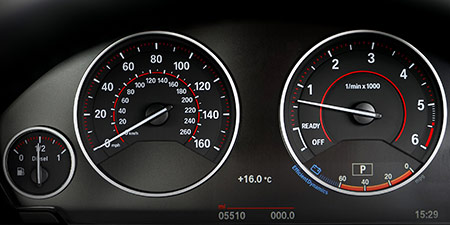# Meters per second (m/s) to Feet per second (ft/s)

Thema Units of speed ﹣ Converter Meters per second to Feet per second

Here you can convert the Speed unit Meters per second into the unit Feet per second and vice versa you can convert Feet per second into Meters per second. By clicking the "Swap units" icon, you will always obtain the desired conversion in the calculation result, i.e., m/s to ft/s or ft/s to m/s. With the following calculator you can also calculate any other Speed unit.

## Info about "Meters per second"

Meters per second is a basic unit for speed in the International System of Units (SI) and other metric systems. This is because both meter and second are the international base units for distance and time. Definition of "meters per second": An object moving at 1 "m/s" per second will cover a distance of one meter.

In our daily work, speed is commonly measured in km/h. The following rule is an easy way to remember when converting m/s into km/h: &qquot; multiply by four and minus 10 %". For example, standard walking speeds in meters per second are about 1 m/s for a walker, 10.44 m/s is the world record for running 100 meters, and the speed of sound is 343 meters per second.

As mentioned above, both meter and second are the international base units for distance and time. All other speed units in the metric system are derived based on meters per second (e.g. km/h, mm/s).

## Info about "Feet per second"

The unit of length in feet is also very common in the Anglo-American countries, therefore speeds are also represented with feet per unit of time. In history, foot is equivalent to 28-32 cm in different countries. Nowadays only the English foot is used. 1 foot is equivalent to 30.48 centimeters and 1 foot is equivalent to 12 inches/inch.

p> It is defined as follows: An object moving at 1 "feet per second"for one second covers a distance of one feet. Therefore, 1 feet is equivalent to 30.48 centimeters.

## Basis for conversion Meters per second (m/s) to Feet per second (ft/s) and vice versa

The abbreviation for the "Speed unit Meters per second" is m/s. The abbreviation for the "Speed unit Feet per second" is ft/s.

## Formula for the conversion of Meters per second (m/s) to Feet per second (ft/s) and vice versa

The calculation from Meters per second to Feet per second shall be made using the following conversion formula:

Conversion formula Meters per second to Feet per second

Determine the number of Feet per second from Meters per second

Meters per second × 3.2808398950131

## Formula for the conversion of Feet per second (ft/s) to Meters per second (m/s)

The calculation from Feet per second to Meters per second shall be made using the following conversion formula:

Conversion formula Feet per second to Meters per second

Determine the number of Meters per second from Feet per second

Feet per second × 0.3048

## Overview table : How many Meters per second are in Feet per second ?

Meters per second m/s ⇒ Feet per second ft/s
0.01 m/s  are  0.03280 ft/s
0.02 m/s  are  0.06561 ft/s
0.03 m/s  are  0.09842 ft/s
0.04 m/s  are  0.13123 ft/s
0.05 m/s  are  0.16404 ft/s
0.06 m/s  are  0.19685 ft/s
0.07 m/s  are  0.22965 ft/s
0.08 m/s  are  0.26246 ft/s
0.09 m/s  are  0.29527 ft/s
0.10 m/s  are  0.32808 ft/s
0.20 m/s  are  0.65616 ft/s
0.30 m/s  are  0.98425 ft/s
0.40 m/s  are  1.31233 ft/s
0.50 m/s  are  1.64042 ft/s
0.60 m/s  are  1.96850 ft/s
0.70 m/s  are  2.29658 ft/s
0.80 m/s  are  2.62467 ft/s
0.90 m/s  are  2.95275 ft/s
1 m/s  corresponds to  3.28084 ft/s
2 m/s  are  6.56168 ft/s
3 m/s  are  9.84252 ft/s
4 m/s  are  13.12336 ft/s
5 m/s  are  16.40419 ft/s
6 m/s  are  19.68503 ft/s
7 m/s  are  22.96587 ft/s
8 m/s  are  26.24671 ft/s
9 m/s  are  29.52755 ft/s
10 m/s  are  32.80839 ft/s
20 m/s  are  65.61679 ft/s
30 m/s  are  98.42519 ft/s
40 m/s  are  131.23359 ft/s
50 m/s  are  164.04199 ft/s
60 m/s  are  196.85039 ft/s
70 m/s  are  229.65879 ft/s
80 m/s  are  262.46719 ft/s
90 m/s  are  295.27559 ft/s
100 m/s  are  328.08399 ft/s
200 m/s  are  656.16797 ft/s
300 m/s  are  984.25196 ft/s
400 m/s  are  1 312.33595 ft/s
500 m/s  are  1 640.41994 ft/s
600 m/s  are  1 968.50393 ft/s
700 m/s  are  2 296.58792 ft/s
800 m/s  are  2 624.67191 ft/s
900 m/s  are  2 952.75590 ft/s
1 000 m/s  are  3 280.83989 ft/s

## Overview table : How many Feet per second are in Meters per second ?

Feet per second ft/s ⇒ Meters per second m/s
0.01 ft/s  are  0.00304 m/s
0.02 ft/s  are  0.00609 m/s
0.03 ft/s  are  0.00914 m/s
0.04 ft/s  are  0.01219 m/s
0.05 ft/s  are  0.01524 m/s
0.06 ft/s  are  0.01828 m/s
0.07 ft/s  are  0.02133 m/s
0.08 ft/s  are  0.02438 m/s
0.09 ft/s  are  0.02743 m/s
0.10 ft/s  are  0.03048 m/s
0.20 ft/s  are  0.06096 m/s
0.30 ft/s  are  0.09144 m/s
0.40 ft/s  are  0.12192 m/s
0.50 ft/s  are  0.15240 m/s
0.60 ft/s  are  0.18288 m/s
0.70 ft/s  are  0.21336 m/s
0.80 ft/s  are  0.24384 m/s
0.90 ft/s  are  0.27432 m/s
1 ft/s  corresponds to  0.30480 m/s
2 ft/s  are  0.60960 m/s
3 ft/s  are  0.91440 m/s
4 ft/s  are  1.21920 m/s
5 ft/s  are  1.52400 m/s
6 ft/s  are  1.82880 m/s
7 ft/s  are  2.13360 m/s
8 ft/s  are  2.43840 m/s
9 ft/s  are  2.74320 m/s
10 ft/s  are  3.04800 m/s
20 ft/s  are  6.09600 m/s
30 ft/s  are  9.14400 m/s
40 ft/s  are  12.19200 m/s
50 ft/s  are  15.24000 m/s
60 ft/s  are  18.28800 m/s
70 ft/s  are  21.33600 m/s
80 ft/s  are  24.38400 m/s
90 ft/s  are  27.43200 m/s
100 ft/s  are  30.48000 m/s
200 ft/s  are  60.96000 m/s
300 ft/s  are  91.44000 m/s
400 ft/s  are  121.92000 m/s
500 ft/s  are  152.40000 m/s
600 ft/s  are  182.88000 m/s
700 ft/s  are  213.36000 m/s
800 ft/s  are  243.84000 m/s
900 ft/s  are  274.32000 m/s
1 000 ft/s  are  304.80000 m/s

## Source information

As source for the information in the "Units of speed" category, we have used in particular:

## Last update on May 3, 2022

The pages of the "Units of speed" category were last editorially reviewed by Stefan Banse on May 3, 2022. They all correspond to the current status.

### Previous changes on October 9, 2021

• November 9, 2020: Publication of the Speed converter
• Editorial revision of all texts in this category